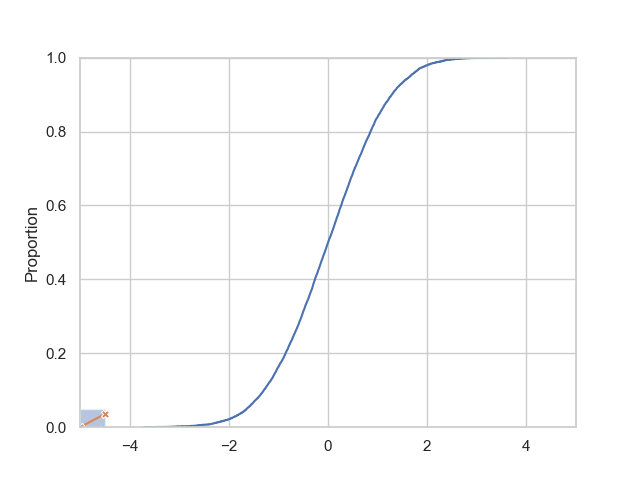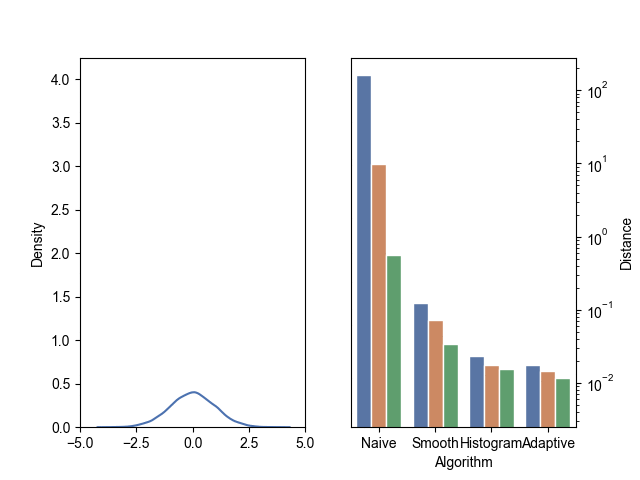Differential privacy in practice

# Estimating a cumulative distribution function with differential privacy

Differential Privacy
Data Science
Clément GastaudSarus builds tools to help compute statistics, analytics, or AI models from sensitive data with formal guarantees of differential privacy.

In this post we will focus on a simple, routine problem: estimating a cumulative distribution function from the observations of a random variable, at the lowest privacy cost possible. It is assumed that the reader knows the basics of differential privacy, nevertheless, we will point to the proper literature when necessary.

As simple as it may sound, this problem is far from trivial when adding the privacy constraint. We will show why by going through four methods of increasing complexity and benchmarking them.

## Formalization of the problem

Our objective is to find the method that best approximates a cumulative distribution function with DP guarantees. To compare the different approaches, we set the privacy budget and look at the distance between the approximated and true distribution. We will quantify the distance using three different metrics: the Kolmogorov-Smirnov statistic, the Wasserstein distance, and the energy distance.

The Kolmogorov-Smirnov statistic is simply the maximal difference between the two functions. Instead of taking the max, the (1-)Wasserstein distance is the integral of the absolute value of the difference between the CDFs:

Note that, in general, the 1-Wasserstein distance is defined as the earth mover’s distance, but in this special case it reduces to this simple form.

Relatedly, the energy distance is:

which penalizes more large deviations between the two distributions.

We search for algorithms that minimize these distances for a given privacy budget.

To keep track of the spent budget, we use the moment’s accountant method introduced by Abadi et al., which gives better bounds than the simple and advanced composition theorems when running several queries with the Gaussian mechanism as it is the case here.

## Description of the methods tested

As an overview of the problem, we propose four simple methods to estimate a CDF. The first three are quite straightforward, while the last one builds on the previous methods.

The most straight-forward method is simply to apply the Gaussian mechanism to evenly-spaced quantiles to get noisy values, and reconstruct the CDF from them. In the first method, we will estimate the noise magnitude using global sensitivity. We will then refine it with smooth sensitivity in the second method.

To further reduce sensitivity, we can opt for an histogram query, which scans uniformly through the given space to evaluate the CDF. This is what we do in the third method.

Finally, we will add adaptivity to the histogram approach to refine the information gained at each privacy leak.

For each approach, we will compare performances on a range of epsilons.

To do so, we generate a dataset X of N = 10,000 samples from a normal distribution and compute the reconstruction error for each epsilon at δ=1/N. Since all exposed methods require the search space to be bounded, we clip all samples to [-5, 5].

### The naive approach: the Gaussian mechanism

First, let’s compute all quantiles independently: each quantile q can be evaluated using the Gaussian mechanism:

with v(q) the true value of the quantile and z Gaussian noise calibrated to the sensitivity of the query. We can then clip the w(q) to [-5, 5] and reorder the values if they happen to be inverted as post-processing.

The sensitivity measures how much the returned value of a query would change if one observation in the dataset was changed. The most conservative approach, global sensitivity, computes the “worst case” value independently of the dataset:

In the case of a quantile, it is the size of the search space since for each quantile q, if we have q points with value -5 and (1-q) with value 5, moving one point would move the quantile’s value from -5 to 5. In order to get a privacy loss of (ε, δ) for one quantile, the added noise should thus be taken from a normal distribution of standard deviation:

This approach gives us the following results:

It clearly does not provide accurate results: simply taking a uniform distribution on the interval would actually approximate the CDF better! This is due to the high sensitivity of the query: for (ε, δ) = (1, 10⁻⁴) on an interval of length 10, we have to add noise with a standard deviation of 188!

### Smooth sensitivity

Since global sensitivity doesn’t make the cut, let’s try to refine it with the smooth sensitivity approach of Nissim et al.. Instead of calculating a worst case scenario of the query independently of the dataset, the noise is calibrated to the variability of the query in the neighborhood of the dataset X. Ideally, we would like add noise proportional to the local sensitivity

with q the index of the element of X closest to the quantile and x[q-1] and x[q+1] the elements closest to x[q] in value. In this framework two neighboring datasets seem at first indistinguishable. However, the magnitude of the added noise would reveal information on the dataset: for example, if x[q-1] = x[q+1]=x[q], the noise added would be 0. To retain indistinguishability, noise magnitude should not only keep track of the neighboring datasets of X, but also those within Hamming distance of 2, 3, …

To do so, Nissim et al. define the β-smooth sensitivity of a query f by:

For quantiles, this can be written as:

With this sensitivity, we can obtain a much closer approximation of the CDF for ε = 1:

This algorithm has a complexity of O(n²), which can be quite time-consuming since datasets can have from a few thousands to several million samples.

In order to improve the accuracy as well as the time overhead, we investigate in the next section a method that circumvents the sensitivity issue.

### Histogram queries

Performance can be improved by simply splitting the space uniformly into bins. Counting the number of observations in a bin is fairly easy since each observation can only affect the counts by 1, thus bounding the sensitivity. Once we have computed the noisy counts for each bin, we can estimate the corresponding quantiles non-privately.

We do not know a priori which quantiles we will get since they are computed from their values and not the other way around. Nevertheless, this is clearly sufficient in order to approximate the CDF.

As we can see, for ε >= 0.1, we get decent accuracy.

The caveat is that the privacy loss increases with the number of bins. Since we are dividing the space uniformly, the estimation is suboptimal for distributions that are very peaked.

A better way to go would be to use the information given by known quantiles to search for the next ones: instead of computing all the bins at the beginning, we can estimate quantiles one by one and proceed by dichotomy to scan the space effectively.

Let’s sketch an algorithm:

• First, initialize a dictionary Q that will store our quantiles: Q = {0: a, 1: b}
• Pick an initial value : xₒ = (a+b) / 2
• Count the number of samples above and below xₒ and add noise
• Deduce the associated quantile q and add it to Q: Q[q] = xₒ
• Repeat with xᵢ chosen as the middle of the largest interval between the q’s until a set privacy budget is spent.

This way, we can be sure that the quantiles are only estimated at the most informative points. In particular, even in the case of a very peaked distribution, we will converge exponentially fast to its high density region regardless of the starting point !

By using an adaptive approach, we manage to increase even more the precision of the reconstructed CDF. As we will see in the next section, this method is also more resilient to highly concentrated distributions.

However, decent results are still hard to achieve for very small ε, at least for this number of observations.

## Analysis and comparison

Let’s now compare these approaches for different distributions. In particular, to explicit the importance of the distribution’s scale, we sample from normal distributions of standard deviations between 1 and 0.01 and plot their performances for (ε=1, δ=1/N):Wasserstein, energy and Kolmogorov-Smirnov distances between true and reconstructed CDFs for gaussian distributions of different standard deviations.

As we can see, the naive approach just does not cut it. Both histogram algorithms give reasonable results, with the adaptive approach reducing the distance by a factor around 2 for reasonably well set bounds. But if the bounds are much further apart or if the standard deviation increases, simple histograms can’t keep up !

In the above figure, we performed a grid search to find the best hyper-parameters (number of quantiles or bins). In practice, this costs privacy which is not reported on the graph, since we have to repeat the experiment for each new hyper-parameter. For epsilon = 1, Here are the performances of each algorithm for several values of the number of bins:

Whatever the number of bins, the adaptive model performs better. Moreover, the range of values that are close to optimal is much larger which makes the algorithm easier to use in practice.

## Conclusion

In this post, we presented a simple mechanism to compute the representation of an arbitrary CDF with good privacy loss. This result is relatively insensitive to hyper-parameters (here the number of quantiles computed and the bounds of the search space). This is especially important in the context of differential privacy since hyper-parameter search is not free.
More elaborate methods are of course possible. For instance, the Propose-Test-Release framework has been proposed for similar objectives. Promising results could also be obtained using convex optimization, an issue that has been extensively studied in the literature (Iyengar et al., Wu et al.) or inverse sensitivity (Asi et al.), which makes use of the exponential mechanism.You're on the list! Thank you for signing up.
Oops! Something went wrong while submitting the form.32, rue Alexandre Dumas
75011 Paris — France
Blog